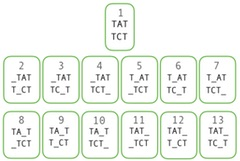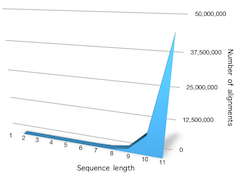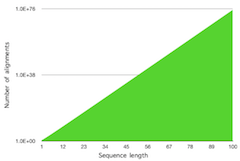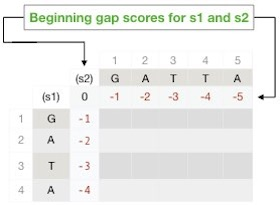# Bioinformatics tutorial: Pair-wise sequence alignment methods 2022

Prerequisites: Knowledge equivalent to the tutorial Pair-wise sequence alignment.
Level: Intermediate.
Learning objectives:
- Gain a basic understanding of Dynamic Programming method for sequence alignment.
- Able to implement a pair-wise global sequence alignment algorithm (Needleman-Wunch).
- Able to implement a local sequence alignment algorithm (Smith-Waterman).

## Introduction

Although we could construct very short and similar sequence alignments by hand, there is no point to do this, since many sequence alignment software tools are available. This tutorial describes the core pair-wise sequence alignment algorithms, consisting of two categories: (1) Global sequence alignments algorithms and (2) Local sequence alignment algorithms.

The first part of this tutorial describes accurate methods, and in the second part, we go through the heuristic approaches of the global and local sequence alignments. Loosely speaking, heuristics means a best guess. Heuristic methods have become necessary, already a long time ago, due to the humongous amount of sequences scientists must handle.

How to find the best alignment between two sequences? To efficiently solve problems, we usually break a large problem into smaller sub-problems. We use the same approach here. In general, to choose the best among a collection of things, we need to compare all of them, and only then we can determine which one is the best of all within the group according to some standard.

Therefore, to be able to choose the best sequence alignment among all possible ones, first, we need to list all possible sequence alignments. Only after that, it is possible to compare all of them and consequently choose the best. However, which one is the best, is determined by a scoring scheme and does not necessarily reflect biological relevance. This tutorial only briefly touches this topic, but you can find an in-depth discussion of this in the related tutorials sub-section below.Figure 1. Two sequence alignments. By allowing gaps with no restriction may result in infinitely long alignments. Gaps are not allowed in the same position in both sequences (b).

Consequently, the next question is how many different sequence alignments can there be between two sequences? Well, it depends on the sequence length and the number of gaps we allow. To begin with, we need to limit their quantity and make a rule that they are not allowed to be in the same position on both of the sequences because the alignments would become infinitely long. Anyway, such sequence alignments would not make sense (Figure 1). Given this constraint, how many different alignments can we then make?Figure 2. By allowing at most one gap, there are 12 possible alignments with gaps and one without gaps. [Click on the image to toggle zoom ◱ ]

Let's make a test with two short sequences of length three. If we don't allow gaps, there is only one possible alignment, since the sequences are the same length. If we permit one gap, there are 12 possible different alignments, given the constraint that no gaps are allowed to be in the same position on both of the sequences. Let's see why. If we insert a gap in the first sequence, there are four different ways we can do that and consequently only three possible positions we can add a gap in the second sequence without making the gaps overlap. Thus, resulting in four times three gapped alignments plus one without gaps, yielding a total of 13 possible sequence alignments (Figure 2).

By following the same logic and allowing precisely two gaps in the first sequence, making it five characters long, we can insert the two gaps in ten different ways, ( 25) = 10. In each of these ten cases, we can add a gap in the second sequence in three different ways. That is, we want to combine two of them; thus, there are three possible ways to do this ( 23) = 3; therefore, we will end up with 30 different alignments (10x3=30).

Similarly, if we insert three gaps in the first sequence, we will have 20 additional alignments. So, the total number of possible alignments of sequences of length three with up to three gaps is 63 (13+30+20=63). Note that we cannot add more than three gaps, because it would result in having only gap characters in the same position on both of the sequences and that is not permitted.Figure 3. The number of possible alignments of sequences up to the length of 11. Both sequences are the same size. [Click on the image to toggle zoom ◱ ]Figure 4. The number of possible alignments of sequences up to the length of 100. Both sequences are the same size. Scale is logarithmic. [Click on the image to toggle zoom ◱ ]

The number of possible sequence alignments increases rapidly with increasing sequence lengths. Two sequences of length 11 have about 45 million possible alignments, and when we grow the length to 134 characters, the number of possible alignments is enormous, about 19x10100. Yes, there are 100 zeros after the number, and it is a googol! Figure 3 shows the number of possible alignments up to the length 11 and Figure 4 shows the number of possible alignments up to 100. Sequences we usually align are much longer than this. For this reason, it is not only impractical but impossible to enumerate all possible alignments; thus, we need to look for smarter algorithms. We explore these in the rest of the tutorial.

Here is a small program that calculates the number of possible sequence alignments for lengths up to 1,000.

## Global sequence alignments

Global sequence alignments are alignments where we align both sequences from start to end. In general, the global approach works best when both sequences are approximately the same length. The local approach may produce alignments entirely containing both sequences, but more about that in the next subsection.

How to go about developing the algorithm? Let's use the approach to break a big problem in smaller sub-problems and consider alignments of one character at a time and then use that information to construct the whole alignment. We use the sequences (s1) GATA and (s2) GATTA and the following simple scoring system: match score = +1, mismatch = -1, and gap = -1.

The following approach describes how to test all possible alignments between s1 and s2. Note that at each character position, we can choose to make (1) a mismatch, (2) a gap in sequence s1, or (3) a gap in s2; thus, we only have three choices at each position.

Before we start the calculations, we make a matrix and put sequence s1 in the first column and s2 in the first row. Then we precompute the gap scores for the column zero from 0 to -5, corresponding to the following positions of s2:
(0) GATTA,
(-1) _ GATTA,
(-2) _ _ GATTA,
(-3) _ _ _ GATTA,
(-4) _ _ _ _ GATTA,
(-5) _ _ _ _ _ GATTA,
and for the sequence s1 correspondingly. Note that we cannot add more than four beginning gaps to the s2 since s1 is only four characters long. By pre-computing the beginning gaps, there is no need to compute them each time we need them (Figure 5).Figure 5. Beginning gap scores (penalties) calculated for sequence s1 and s2 for the alignment calculations at the first row and column. The penalties for s1 are in the column to the right of s1 and for s2 in the row directly under the sequence.